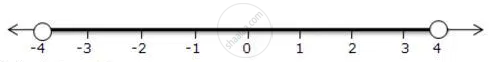Share

Books Shortlist

# Represent the Following Inequalities on Real Number Lines -4 < X < 4 - ICSE Class 10 - Mathematics

ConceptLinear Inequations in One Unknown

#### Question

Represent the following inequalities on real number lines

-4 < x < 4

#### Solution

-4 < x < 4

Solution on number line isIs there an error in this question or solution?

#### APPEARS IN

Solution Represent the Following Inequalities on Real Number Lines -4 < X < 4 Concept: Linear Inequations in One Unknown.
S